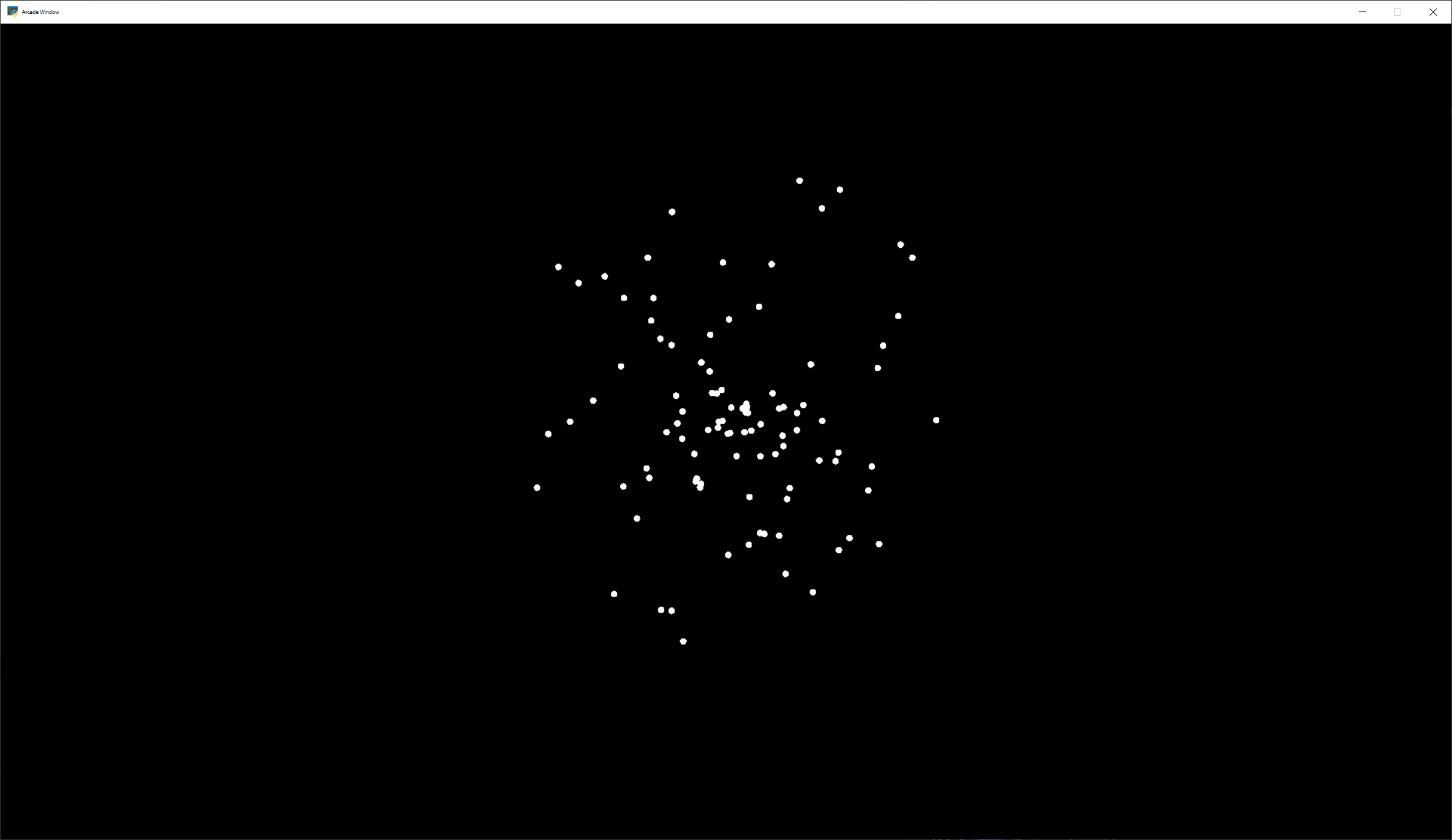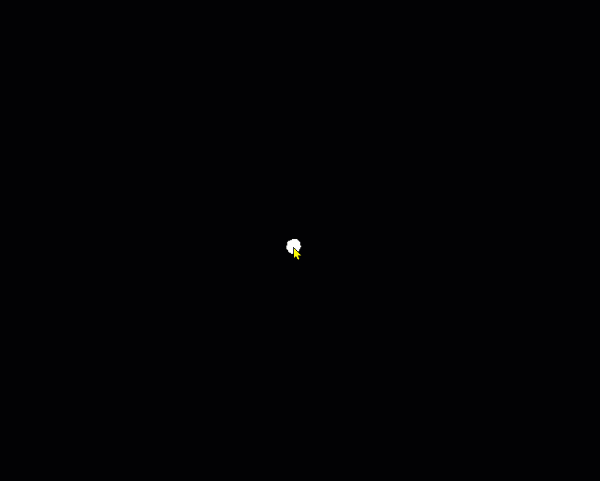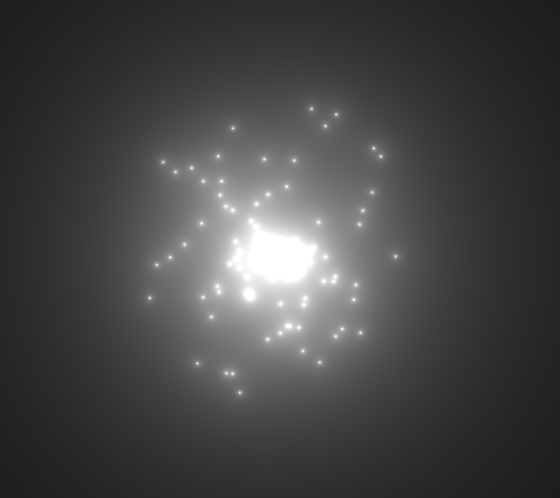This tutorial assumes you are already familiar with the material in Shader Toy - Glow. In this tutorial, we take a look at adding animated particles. These particles can be used for an explosion effect.

The “trick” to this example, is the use of pseudo-random numbers to generate each particle’s angle and speed from the initial explosion point. Why “pseudo-random”? This allows each processor on the GPU to independently calculate each particle’s position at any point and time. We can then allow the GPU to calculate in parallel.

First, we need a program that will load a shader. This program is also keeping track of how much time has elapsed. This is necessary for us to calculate how far along the animation sequence we are.

``` 1import arcade
3
4
5# Derive an application window from Arcade's parent Window class
7
8    def __init__(self):
9        # Call the parent constructor
10        super().__init__(width=1920, height=1080)
11
12        # Used to track run-time
13        self.time = 0.0
14
16        file_name = "explosion.glsl"
19
20    def on_draw(self):
21        self.clear()
22        # Set uniform data to send to the GLSL shader
24
25        # Run the GLSL code
27
28    def on_update(self, delta_time: float):
29        # Track run time
30        self.time += delta_time
31
32
33if __name__ == "__main__":
34    window = MyGame()
35    window.center_window()
`````` 1// Origin of the particles
2uniform vec2 pos;
3
4// Constants
5
6// Number of particles
7const float PARTICLE_COUNT = 100.0;
8// Max distance the particle can be from the position.
9// Normalized. (So, 0.3 is 30% of the screen.)
10const float MAX_PARTICLE_DISTANCE = 0.3;
11// Size of each particle. Normalized.
12const float PARTICLE_SIZE = 0.004;
13const float TWOPI = 6.2832;
14
15// This function will return two pseudo-random numbers given an input seed.
16// The result is in polar coordinates, to make the points random in a circle
17// rather than a rectangle.
18vec2 Hash12_Polar(float t) {
19  float angle = fract(sin(t * 674.3) * 453.2) * TWOPI;
20  float distance = fract(sin((t + angle) * 724.3) * 341.2);
21  return vec2(sin(angle), cos(angle)) * distance;
22}
23
24void mainImage( out vec4 fragColor, in vec2 fragCoord )
25{
26    // Normalized pixel coordinates (from 0 to 1)
27    // Origin of the particles
28    vec2 npos = (pos - .5 * iResolution.xy) / iResolution.y;
29    // Position of current pixel we are drawing
30    vec2 uv = (fragCoord- .5 * iResolution.xy) / iResolution.y;
31
32    // Re-center based on input coordinates, rather than origin.
33    uv -= npos;
34
35    // Default alpha is transparent.
36    float alpha = 0.0;
37
38    // Loop for each particle
39    for (float i= 0.; i < PARTICLE_COUNT; i++) {
40        // Direction of particle + speed
41        float seed = i + 1.0;
42        vec2 dir = Hash12_Polar(seed);
43        // Get position based on direction, magnitude, and explosion size
44        vec2 particlePosition = dir * MAX_PARTICLE_DISTANCE;
45        // Distance of this pixel from that particle
46        float d = length(uv - particlePosition);
47        // If we are within the particle size, set alpha to 1.0
48        if (d < PARTICLE_SIZE)
49            alpha = 1.0;
50    }
51    // Output to screen
52    fragColor = vec4(1.0, 1.0, 1.0, alpha);
53}
`````` 1// Origin of the particles
2uniform vec2 pos;
3
4// Constants
5
6// Number of particles
7const float PARTICLE_COUNT = 100.0;
8// Max distance the particle can be from the position.
9// Normalized. (So, 0.3 is 30% of the screen.)
10const float MAX_PARTICLE_DISTANCE = 0.3;
11// Size of each particle. Normalized.
12const float PARTICLE_SIZE = 0.004;
13// Time for each burst cycle, in seconds.
14const float BURST_TIME = 2.0;
15const float TWOPI = 6.2832;
16
17// This function will return two pseudo-random numbers given an input seed.
18// The result is in polar coordinates, to make the points random in a circle
19// rather than a rectangle.
20vec2 Hash12_Polar(float t) {
21  float angle = fract(sin(t * 674.3) * 453.2) * TWOPI;
22  float distance = fract(sin((t + angle) * 724.3) * 341.2);
23  return vec2(sin(angle), cos(angle)) * distance;
24}
25
26void mainImage( out vec4 fragColor, in vec2 fragCoord )
27{
28    // Normalized pixel coordinates (from 0 to 1)
29    // Origin of the particles
30    vec2 npos = (pos - .5 * iResolution.xy) / iResolution.y;
31    // Position of current pixel we are drawing
32    vec2 uv = (fragCoord- .5 * iResolution.xy) / iResolution.y;
33
34    // Re-center based on input coordinates, rather than origin.
35    uv -= npos;
36
37    // Default alpha is transparent.
38    float alpha = 0.0;
39
40    // 0.0 - 1.0 normalized fraction representing how far along in the explosion we are.
41    // Auto resets if time goes beyond burst time. This causes the explosion to cycle.
42    float timeFract = fract(iTime * 1 / BURST_TIME);
43
44    // Loop for each particle
45    for (float i= 0.; i < PARTICLE_COUNT; i++) {
46        // Direction of particle + speed
47        float seed = i + 1.0;
48        vec2 dir = Hash12_Polar(seed);
49        // Get position based on direction, magnitude, and explosion size
50        // Adjust based on time scale. (0.0-1.0)
51        vec2 particlePosition = dir * MAX_PARTICLE_DISTANCE * timeFract;
52        // Distance of this pixel from that particle
53        float d = length(uv - particlePosition);
54        // If we are within the particle size, set alpha to 1.0
55        if (d < PARTICLE_SIZE)
56            alpha = 1.0;
57    }
58    // Output to screen
59    fragColor = vec4(1.0, 1.0, 1.0, alpha);
60}
```

``` 1// Origin of the particles
2uniform vec2 pos;
3
4// Constants
5
6// Number of particles
7const float PARTICLE_COUNT = 100.0;
8// Max distance the particle can be from the position.
9// Normalized. (So, 0.3 is 30% of the screen.)
10const float MAX_PARTICLE_DISTANCE = 0.3;
11// Size of each particle. Normalized.
12const float PARTICLE_SIZE = 0.004;
13// Time for each burst cycle, in seconds.
14const float BURST_TIME = 2.0;
15const float TWOPI = 6.2832;
16
17// This function will return two pseudo-random numbers given an input seed.
18// The result is in polar coordinates, to make the points random in a circle
19// rather than a rectangle.
20vec2 Hash12_Polar(float t) {
21  float angle = fract(sin(t * 674.3) * 453.2) * TWOPI;
22  float distance = fract(sin((t + angle) * 724.3) * 341.2);
23  return vec2(sin(angle), cos(angle)) * distance;
24}
25
26void mainImage( out vec4 fragColor, in vec2 fragCoord )
27{
28    // Normalized pixel coordinates (from 0 to 1)
29    // Origin of the particles
30    vec2 npos = (pos - .5 * iResolution.xy) / iResolution.y;
31    // Position of current pixel we are drawing
32    vec2 uv = (fragCoord- .5 * iResolution.xy) / iResolution.y;
33
34    // Re-center based on input coordinates, rather than origin.
35    uv -= npos;
36
37    // Default alpha is transparent.
38    float alpha = 0.0;
39
40    // 0.0 - 1.0 normalized fraction representing how far along in the explosion we are.
41    // Auto resets if time goes beyond burst time. This causes the explosion to cycle.
42    float timeFract = fract(iTime * 1 / BURST_TIME);
43
44    // Loop for each particle
45    for (float i= 0.; i < PARTICLE_COUNT; i++) {
46        // Direction of particle + speed
47        float seed = i + 1.0;
48        vec2 dir = Hash12_Polar(seed);
49        // Get position based on direction, magnitude, and explosion size
50        // Adjust based on time scale. (0.0-1.0)
51        vec2 particlePosition = dir * MAX_PARTICLE_DISTANCE * timeFract;
52        // Distance of this pixel from that particle
53        float d = length(uv - particlePosition);
54        // If we are within the particle size, set alpha to 1.0
55        if (d < PARTICLE_SIZE)
56            alpha = 1.0;
57    }
58    // Output to screen
59    fragColor = vec4(1.0, 1.0, 1.0, alpha * (1.0 - timeFract));
60}
```

## Glowing Particles#``` 1// Origin of the particles
2uniform vec2 pos;
3
4// Constants
5
6// Number of particles
7const float PARTICLE_COUNT = 100.0;
8// Max distance the particle can be from the position.
9// Normalized. (So, 0.3 is 30% of the screen.)
10const float MAX_PARTICLE_DISTANCE = 0.3;
11// Size of each particle. Normalized.
12const float PARTICLE_SIZE = 0.004;
13// Time for each burst cycle, in seconds.
14const float BURST_TIME = 2.0;
15// Particle brightness
16const float DEFAULT_BRIGHTNESS = 0.0005;
17
18const float TWOPI = 6.2832;
19
20// This function will return two pseudo-random numbers given an input seed.
21// The result is in polar coordinates, to make the points random in a circle
22// rather than a rectangle.
23vec2 Hash12_Polar(float t) {
24  float angle = fract(sin(t * 674.3) * 453.2) * TWOPI;
25  float distance = fract(sin((t + angle) * 724.3) * 341.2);
26  return vec2(sin(angle), cos(angle)) * distance;
27}
28
29void mainImage( out vec4 fragColor, in vec2 fragCoord )
30{
31    // Normalized pixel coordinates (from 0 to 1)
32    // Origin of the particles
33    vec2 npos = (pos - .5 * iResolution.xy) / iResolution.y;
34    // Position of current pixel we are drawing
35    vec2 uv = (fragCoord- .5 * iResolution.xy) / iResolution.y;
36
37    // Re-center based on input coordinates, rather than origin.
38    uv -= npos;
39
40    // Default alpha is transparent.
41    float alpha = 0.0;
42
43    // 0.0 - 1.0 normalized fraction representing how far along in the explosion we are.
44    // Auto resets if time goes beyond burst time. This causes the explosion to cycle.
45    float timeFract = fract(iTime * 1 / BURST_TIME);
46
47    // Loop for each particle
48    for (float i= 0.; i < PARTICLE_COUNT; i++) {
49        // Direction of particle + speed
50        float seed = i + 1.0;
51        vec2 dir = Hash12_Polar(seed);
52        // Get position based on direction, magnitude, and explosion size
53        // Adjust based on time scale. (0.0-1.0)
54        vec2 particlePosition = dir * MAX_PARTICLE_DISTANCE * timeFract;
55        // Distance of this pixel from that particle
56        float d = length(uv - particlePosition);
57        // Add glow based on distance
58        alpha += DEFAULT_BRIGHTNESS / d;
59    }
60    // Output to screen
61    fragColor = vec4(1.0, 1.0, 1.0, alpha * (1.0 - timeFract));
62}
```

## Twinkling Particles#

``` 1// Origin of the particles
2uniform vec2 pos;
3
4// Constants
5
6// Number of particles
7const float PARTICLE_COUNT = 100.0;
8// Max distance the particle can be from the position.
9// Normalized. (So, 0.3 is 30% of the screen.)
10const float MAX_PARTICLE_DISTANCE = 0.3;
11// Size of each particle. Normalized.
12const float PARTICLE_SIZE = 0.004;
13// Time for each burst cycle, in seconds.
14const float BURST_TIME = 2.0;
15// Particle brightness
16const float DEFAULT_BRIGHTNESS = 0.0005;
17// How many times to the particles twinkle
18const float TWINKLE_SPEED = 10.0;
19
20const float TWOPI = 6.2832;
21
22// This function will return two pseudo-random numbers given an input seed.
23// The result is in polar coordinates, to make the points random in a circle
24// rather than a rectangle.
25vec2 Hash12_Polar(float t) {
26  float angle = fract(sin(t * 674.3) * 453.2) * TWOPI;
27  float distance = fract(sin((t + angle) * 724.3) * 341.2);
28  return vec2(sin(angle), cos(angle)) * distance;
29}
30
31void mainImage( out vec4 fragColor, in vec2 fragCoord )
32{
33    // Normalized pixel coordinates (from 0 to 1)
34    // Origin of the particles
35    vec2 npos = (pos - .5 * iResolution.xy) / iResolution.y;
36    // Position of current pixel we are drawing
37    vec2 uv = (fragCoord- .5 * iResolution.xy) / iResolution.y;
38
39    // Re-center based on input coordinates, rather than origin.
40    uv -= npos;
41
42    // Default alpha is transparent.
43    float alpha = 0.0;
44
45    // 0.0 - 1.0 normalized fraction representing how far along in the explosion we are.
46    // Auto resets if time goes beyond burst time. This causes the explosion to cycle.
47    float timeFract = fract(iTime * 1 / BURST_TIME);
48
49    // Loop for each particle
50    for (float i= 0.; i < PARTICLE_COUNT; i++) {
51        // Direction of particle + speed
52        float seed = i + 1.0;
53        vec2 dir = Hash12_Polar(seed);
54        // Get position based on direction, magnitude, and explosion size
55        // Adjust based on time scale. (0.0-1.0)
56        vec2 particlePosition = dir * MAX_PARTICLE_DISTANCE * timeFract;
57        // Distance of this pixel from that particle
58        float d = length(uv - particlePosition);
59        // Add glow based on distance
60        float brightness = DEFAULT_BRIGHTNESS * (sin(timeFract * TWINKLE_SPEED + i) * .5 + .5);
61        alpha += brightness / d;
62    }
63    // Output to screen
64    fragColor = vec4(1.0, 1.0, 1.0, alpha * (1.0 - timeFract));
65}
```